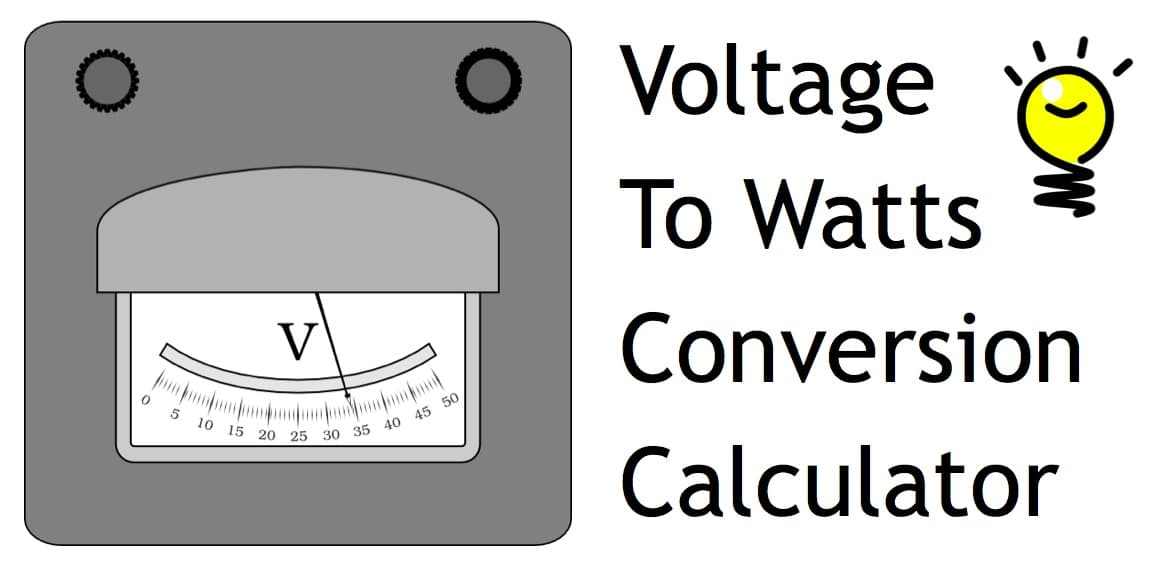# Volts to Watts, Watts to Amps, Volts to Amps Conversion Calculator

Our online calculator / conversion tool can convert watts to amps, volts to watts, and volts to amps. The calculator works by filling in any of two of the three fields (volts amps watts) to calculate the value of the third field. This tool can convert any value as long as you enter the two other values.

Voltage:
Watts:
Amps:

## Example Conversions

Example 1: To convert volts to amps for a 24V VA50 power supply, enter 24 volts and 50 Watts. Click Calculate.

Example 2: To convert watts to amps for a 12V DC 500mA power supply, enter 12 volts and .5 amps. Click Calculate.

1. How do you convert volts to watts?
The formula to convert voltage to watts is watts = amps x volts.
2. How do you convert watts to amps?
The formula to convert watts to amps at a fixed voltage is amps = watts / volts.
3. How do you convert volts to amps?
The formula to convert volts to amps at a fixed wattage is: amps = watts / volts.
4. How do you convert amps to watts?
The formula to convert amps to watts at a fixed voltage is: watts = amps x volts.

## Convert Watts to Amps (Detailed Example)

Here is one example of how this calculator is commonly used by security system installers as an amp calculator. The installer needs to calculate the distance that a power cable can be run from a surveillance DVR to a CCTV camera, HD security camera, and even one of the latest UHD 4K security cameras. They first need to calculate how many amps a 24V AC power supply outputs. Typically, 24V AC power supplies have VA (voltage amps) ratings and not amps. For example a 24VAC50 power supply is 24 volt, 50 volt-amps (watts are also known as volt-amps). In the above calculator, the installer will enter a value of 24 in the volts field and a value of 50 in the watts field.

## Electrical Term Definitions

Here are some useful electrical terms related to calculating volts to watts, watts to amps, and volts to amps.

• Volt – unit of measure of the electrical force or pressure which causes an electricity current to flow in a circuit. One volt is the amount of pressure required to cause one ampere of current to flow against one ohm of resistance. The concept is similar to water pressure.
• Watt – unit of measure of the electrical power applied in a circuit. Watts are also known as volt-amps and are a unit of electrical measurement typically used in AC power circuits. Watts are calculated by multiplying the current (measured in amps) by the electrical pressure (measured in volts).
• Amp (Ampere) – unit of measure of the flow rate of current in an electrical circuit. One amp is the amount of current when one volt of electrical pressure is applied against one ohm of resistance. Amps are used to measure electricity flow similarly to how GPM (gallons per minute) are used to measure the volume of water flowing.
• Ohm – unit for measuring the resistance of flow in an electrical current. Electrical conductors (such as a wire) apply resistance to the flow of current. This is similar to how a tube or hose applies resistance to the flow of water. One ohm is the amount of resistance that limits current flow to one amp in a circuit with one volt of electrical pressure.
• Ohm’s Law – Ohm’s Law states that when electric current flows through a conductor (such as a cable), the intensity of the current (amps) equals the electromotive force (volts) driving it, divided by the resistance of the conductor.